An ice-making machine inside a refrigerator operates in a Carnot cycle. It takes heat from liquid water at 0.0 degrees Celsius and rejects h

Question

An ice-making machine inside a refrigerator operates in a Carnot cycle. It takes heat from liquid water at 0.0 degrees Celsius and rejects heat to a room at a temperature of 20.6 degrees Celsius. Suppose that liquid water with a mass of 82.1 kg at 0.0 degrees Celsius is converted to ice at the same temperature. Take the heat of fusion for water to be Lf=3.34×105 J/kg.
A. How much heat |QH| is rejected to the room?
B. How much energy E must be supplied to the device?

in progress 0
5 months 2021-08-04T06:51:08+00:00 1 Answers 7 views 0

A. Q = 2.74 x 10⁷ J = 27.4 MJ

B. E = 3.91 x 10⁸ J = 391 MJ

Explanation:

A.

Heat rejected can be found as follows: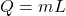where,

Q = Heat rejected = ?

m = mass = 82.1 kg

L = Latent Heat of fusion = 334000 J/kg

Therefore,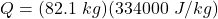Q = 2.74 x 10⁷ J = 27.4 MJ

B.

First, we will calculate the efficiency of the Carnot Cycle as follows: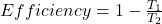where,

T₁ = Heat intake temperature = 0°C + 273 = 273 k

T₂ = Heat rejection temperature = 20.6°C + 273 = 293.6 k

Therefore,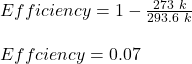Therefore, the energy input required is: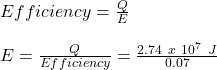E = 3.91 x 10⁸ J = 391 MJ